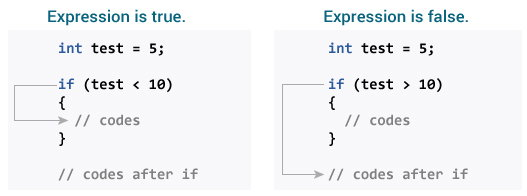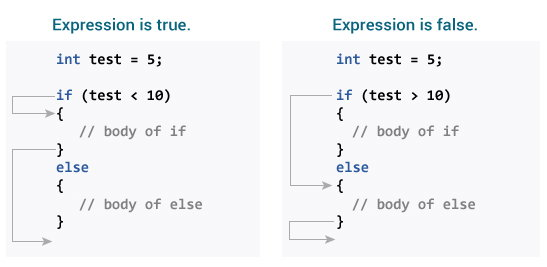C if...else Statement

# C if...else Statement

## C if Statement

The syntax of the `if` statement in C programming is:

``````if (test expression)
{
// statements to be executed if the test expression is true
}``````

### How if statement works?

The `if` statement evaluates the test expression inside the parenthesis `()`.

• If the test expression is evaluated to true, statements inside the body of `if` are executed.
• If the test expression is evaluated to false, statements inside the body of `if` are not executed.To learn more about when test expression is evaluated to true (non-zero value) and false (0), check relational and logical operators.

### Example 1: if statement

``````// Program to display a number if it is negative

#include <stdio.h>
int main() {
int number;

printf("Enter an integer: ");
scanf("%d", &number);

// true if number is less than 0
if (number < 0) {
printf("You entered %d.\n", number);
}

printf("The if statement is easy.");

return 0;
}``````

Output 1

```Enter an integer: -2
You entered -2.
The if statement is easy.```

When the user enters -2, the test expression `number<0` is evaluated to true. Hence, You entered -2 is displayed on the screen.

Output 2

```Enter an integer: 5
The if statement is easy.```

When the user enters 5, the test expression `number<0` is evaluated to false and the statement inside the body of `if` is not executed

## C if...else Statement

The `if` statement may have an optional `else` block. The syntax of the `if..else` statement is:

``````if (test expression) {
// statements to be executed if the test expression is true
}
else {
// statements to be executed if the test expression is false
}``````

### How if...else statement works?

If the test expression is evaluated to true,

• statements inside the body of `if` are executed.
• statements inside the body of `else` are skipped from execution.

If the test expression is evaluated to false,

• statements inside the body of `else` are executed
• statements inside the body of `if` are skipped from execution.### Example 2: if...else statement

``````// Check whether an integer is odd or even

#include <stdio.h>
int main() {
int number;
printf("Enter an integer: ");
scanf("%d", &number);

// True if the remainder is 0
if  (number%2 == 0) {
printf("%d is an even integer.",number);
}
else {
printf("%d is an odd integer.",number);
}

return 0;
}``````

Output

```Enter an integer: 7
7 is an odd integer.```

When the user enters 7, the test expression `number%2==0` is evaluated to false. Hence, the statement inside the body of `else` is executed.

The `if...else` statement executes two different codes depending upon whether the test expression is true or false. Sometimes, a choice has to be made from more than 2 possibilities.

The if...else ladder allows you to check between multiple test expressions and execute different statements.

``````if (test expression1) {
// statement(s)
}
else if(test expression2) {
// statement(s)
}
else if (test expression3) {
// statement(s)
}
.
.
else {
// statement(s)
}``````

### Example 3: C if...else Ladder

``````// Program to relate two integers using =, > or < symbol

#include <stdio.h>
int main() {
int number1, number2;
printf("Enter two integers: ");
scanf("%d %d", &number1, &number2);

//checks if the two integers are equal.
if(number1 == number2) {
printf("Result: %d = %d",number1,number2);
}

//checks if number1 is greater than number2.
else if (number1 > number2) {
printf("Result: %d > %d", number1, number2);
}

//checks if both test expressions are false
else {
printf("Result: %d < %d",number1, number2);
}

return 0;
}``````

Output

```Enter two integers: 12
23
Result: 12 < 23```

## Nested if...else

It is possible to include an `if...else` statement inside the body of another `if...else` statement.

### Example 4: Nested if...else

This program given below relates two integers using either `<`, `>` and `=` similar to the `if...else` ladder's example. However, we will use a nested `if...else` statement to solve this problem.

``````#include <stdio.h>
int main() {
int number1, number2;
printf("Enter two integers: ");
scanf("%d %d", &number1, &number2);

if (number1 >= number2) {
if (number1 == number2) {
printf("Result: %d = %d",number1,number2);
}
else {
printf("Result: %d > %d", number1, number2);
}
}
else {
printf("Result: %d < %d",number1, number2);
}

return 0;
}``````

If the body of an `if...else` statement has only one statement, you do not need to use brackets `{}`.

For example, this code

``````if (a > b) {
print("Hello");
}
print("Hi");``````

is equivalent to

``````if (a > b)
print("Hello");
print("Hi");```
```